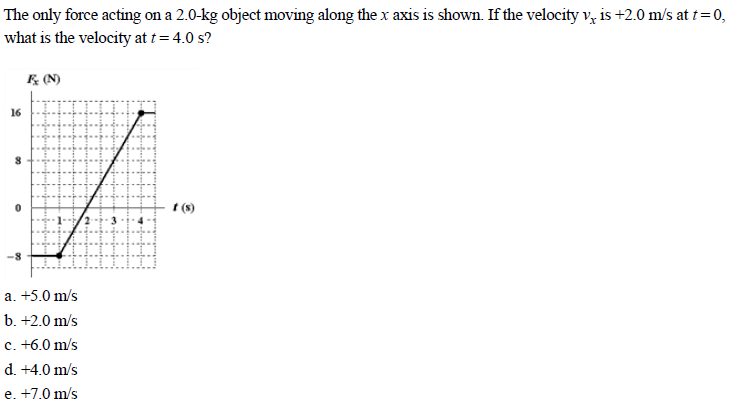# The only force acting on a 2.0-kg object moving along the x axis is shown. If the velocity v is +2.0 m/s at t 0, what is the velocity at t = 4.0 s? R IN 16 - 8 r (s) a. +5.0 m/s b. +2.0 m/s c. +6.0 m/s d. +4.0 m/s e. +7.0 m/s

Questionhelp_outlineImage TranscriptioncloseThe only force acting on a 2.0-kg object moving along the x axis is shown. If the velocity v is +2.0 m/s at t 0, what is the velocity at t = 4.0 s? R IN 16 - 8 r (s) a. +5.0 m/s b. +2.0 m/s c. +6.0 m/s d. +4.0 m/s e. +7.0 m/s fullscreen

### Want to see the step-by-step answer?